# percentile(), percentiles() (aggregation function)

Returns an estimate for the specified nearest-rank percentile of the population defined by `*Expr*`. The accuracy depends on the density of population in the region of the percentile. This function can be used only in context of aggregation inside summarize

• `percentiles()` is like `percentile()`, but calculates a number of percentile values, which is faster than calculating each percentile individually.
• `percentilesw()` is like `percentilew()`, but calculates a number of weighted percentile values, which is faster than calculating each percentile individually.
• `percentilew()` and `percentilesw()` let you calculate weighted percentiles. Weighted percentiles calculate the given percentiles in a "weighted" way, by treating each value as if it was repeated `weight` times, in the input.

To add a percentage calculation to your results, see the percentages example.

## Syntax

`percentile` `(`Expr`,` Percentile`)`

`percentiles` `(`Expr`,` Percentile1 [`,` Percentile2]`)`

`percentiles_array` `(`Expr`,` Percentile1 [`,` Percentile2]`)`

`percentiles_array` `(`Expr`,` Dynamic array`)`

`percentilew` `(`Expr`,` WeightExpr`,` Percentile`)`

`percentilesw` `(`Expr`,` WeightExpr`,` Percentile1 [`,` Percentile2]`)`

`percentilesw_array` `(`Expr`,` WeightExpr`,` Percentile1 [`,` Percentile2]`)`

`percentilesw_array` `(`Expr`,` WeightExpr`,` Dynamic array`)`

## Arguments

• Expr: Expression that will be used for aggregation calculation.
• WeightExpr: Expression that will be used as the weight of values for aggregation calculation.
• Percentile: A double constant that specifies the percentile.
• Dynamic array: list of percentiles in a dynamic array of integer or floating point numbers.

## Returns

Returns an estimate for `*Expr*` of the specified percentiles in the group.

## Examples

The value of `Duration` that is larger than 95% of the sample set and smaller than 5% of the sample set.

``````CallDetailRecords | summarize percentile(Duration, 95) by continent
``````

Simultaneously calculate 5, 50 (median) and 95.

``````CallDetailRecords
| summarize percentiles(Duration, 5, 50, 95) by continent
``````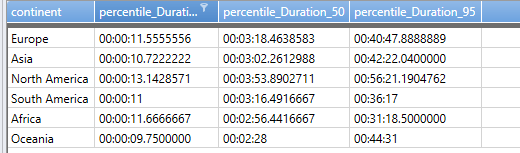The results show that in Europe, 5% of calls are shorter than 11.55s, 50% of calls are shorter than 3 minutes, 18.46 seconds, and 95% of calls are shorter than 40 minutes 48 seconds.

``````CallDetailRecords
| summarize percentiles(Duration, 5, 50, 95), avg(Duration)
``````

## Weighted percentiles

Assume you repetitively measure the time (Duration) it takes an action to complete. Instead of recording every value of the measurement, you record each value of Duration, rounded to 100 msec, and how many times the rounded value appeared (BucketSize).

Use `summarize percentilesw(Duration, BucketSize, ...)` to calculate the given percentiles in a "weighted" way. Treat each value of Duration as if it was repeated BucketSize times in the input, without actually needing to materialize those records.

## Example

A customer has a set of latency values in milliseconds: `{ 1, 1, 2, 2, 2, 5, 7, 7, 12, 12, 15, 15, 15, 18, 21, 22, 26, 35 }`.

To reduce bandwidth and storage, do pre-aggregation to the following buckets: `{ 10, 20, 30, 40, 50, 100 }`. Count the number of events in each bucket to produce the following table: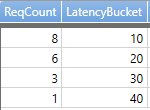The table displays:

• Eight events in the 10-ms bucket (corresponding to subset `{ 1, 1, 2, 2, 2, 5, 7, 7 }`)
• Six events in the 20-ms bucket (corresponding to subset `{ 12, 12, 15, 15, 15, 18 }`)
• Three events in the 30-ms bucket (corresponding to subset `{ 21, 22, 26 }`)
• One event in the 40-ms bucket (corresponding to subset `{ 35 }`)

At this point, the original data is no longer available. Only the number of events in each bucket. To compute percentiles from this data, use the `percentilesw()` function. For example, for the 50, 75, and 99.9 percentiles, use the following query.

``````datatable (ReqCount:long, LatencyBucket:long)
[
8, 10,
6, 20,
3, 30,
1, 40
]
| summarize percentilesw(LatencyBucket, ReqCount, 50, 75, 99.9)
``````

The result for the above query is: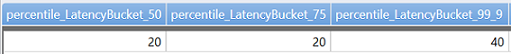The above query corresponds to the function `percentiles(LatencyBucket, 50, 75, 99.9)`, if the data was expanded to the following form: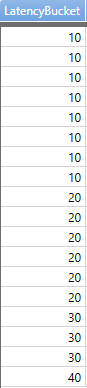## Getting multiple percentiles in an array

Multiple percentiles can be obtained as an array in a single dynamic column, instead of in multiple columns.

``````CallDetailRecords
| summarize percentiles_array(Duration, 5, 25, 50, 75, 95), avg(Duration)
``````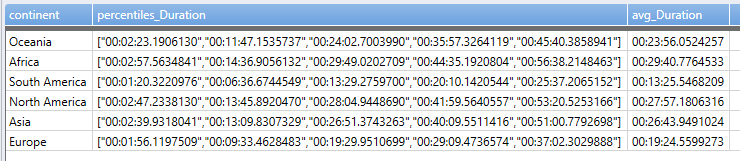Similarly, weighted percentiles can be returned as a dynamic array using `percentilesw_array`.

Percentiles for `percentiles_array` and `percentilesw_array` can be specified in a dynamic array of integer or floating-point numbers. The array must be constant but doesn't have to be literal.

``````CallDetailRecords
| summarize percentiles_array(Duration, dynamic([5, 25, 50, 75, 95])), avg(Duration)
``````
``````CallDetailRecords
| summarize percentiles_array(Duration, range(0, 100, 5)), avg(Duration)
``````

## Nearest-rank percentile

P-th percentile (0 < P <= 100) of a list of ordered values, sorted from least to greatest, is the smallest value in the list. The P percent of the data is less or equal to P-th percentile value (from Wikipedia article on percentiles).

Define 0-th percentiles to be the smallest member of the population.

Note

Given the approximating nature of the calculation, the actual returned value may not be a member of the population. Nearest-rank definition means that P=50 does not conform to the interpolative definition of the median. When evaluating the significance of this discrepancy for the specific application, the size of the population and an estimation error should be taken into account.

## Estimation error in percentiles

The percentiles aggregate provides an approximate value using T-Digest.

Note

• The bounds on the estimation error vary with the value of the requested percentile. The best accuracy is at both ends of the [0..100] scale. Percentiles 0 and 100 are the exact minimum and maximum values of the distribution. The accuracy gradually decreases towards the middle of the scale. It's worst at the median and is capped at 1%.
• Error bounds are observed on the rank, not on the value. Suppose percentile(X, 50) returned a value of Xm. The estimate guarantees that at least 49% and at most 51% of the values of X are less or equal to Xm. There is no theoretical limit on the difference between Xm and the actual median value of X.
• The estimation may sometimes result in a precise value but there are no reliable conditions to define when it will be the case.